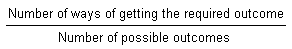HOME MATH DICTIONARY DOWNLOAD FEEDBACK DISCLAIMER
 Question: What is Probability ? Answer: There are many situations where we have an intuitive idea of how likely they are to happen based on past experience. We may, for example, look at the sky and the barometer and decide that there is a good chance that it will rain. Mathematician try to put a number to such an event to give a clearer idea of how likely it is to happen. When an event is a certainty, such as the chance that you will die one day, it is given the number 1. When the chance of an event happening is zero, such as you swimming the Atlantic ocean, then it is given the number 0. tossing a coin to see whether it come up head or tail is used to start many games because the likelihood of either happening is the same, so it is the given number 1/2 .This numerical value of the likelihood of an event happening is called its probability, and it always takes a value between 0 and 1. Generally, the probability of a particular outcome for equally likely events is given byWhen a die is rolled there are six possible outcomes which are all equally likely, so as only one of these gives a 5 uppermost, the probability of scoring a 5 is said to be 1/6 . In a standard pack of 52 playing cards there are 4 kings, so the probability that if you take one card from the pack it will be a king is 4/52 = 1/13. The probability of drawing a prime number from the pack would be 16/52 = 4/13, as there are 16 prime numbers in the pack, namely the 2,3,5 and 7 of clubs, hearts, diamonds and spades Probability is also determined by keeping records of similar events over a long run. For example, a sheep farmer may know from her records that on average one-third of her ewes have twin lamps, so she would give the probability of having twins at each birth as 1/3.